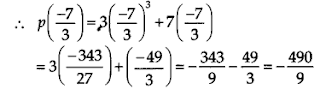NCERT Solutions for Class 9 Maths Chapter 2 Polynomials Ex 2.3

# NCERT Solutions for Class 9 Maths Chapter 2 Polynomials Ex 2.3

## NCERT Solutions for Class 9 Maths Chapter 2 Polynomials Ex 2.3

NCERT Solutions for Class 9 Maths Chapter 2 Polynomials Ex 2.3 are the part of NCERT Solutions for Class 9 Maths. Here you can find the NCERT Solutions for Class 9 Maths Chapter 2 Polynomials Ex 2.3.

Ex 2.3 Class 9 Maths Question 1.
Find the remainder when x3 + 3x2 + 3x + 1 is divided by
(i) x + 1
(ii) x – ½
(iii) x
(iv) x + π
(v) 5 + 2x

Solution:
Let p(x) = x3 + 3x2 + 3x + 1
(i) The zero of x + 1 is -1.
p(-1) = (-1)3 + 3(-1)2 + 3(-1) + 1
= -1 + 3 – 3 + 1 = 0
Thus, the required remainder = 0

(iii) The zero of x is 0.
p(0) = (0)3 + 3(0)2 + 3(0) + 1
= 0 + 0 + 0 + 1 = 1
Thus, the required remainder = 1.

(iv) The zero of x + π is -π.
p(-π) = (-π)3 + 3(-π)2 + 3(-π) + 1
= -π3 + 3π2 + (-3π) + 1
= -π3 + 3π2 – 3π + 1
Thus, the required remainder is -π3 + 3π2 – 3π + 1.

Ex 2.3 Class 9 Maths Question 2.

Find the remainder when x3 – ax2 + 6x – a is divided by x – a.

Solution:
We have, p(x) = x3 – ax2 + 6x – a and zero of x – a is a.
p(a) = (a)3 – a(a)2 + 6(a) – a
= a3 – a3 + 6a – a = 5a
Thus, the required remainder is 5a.

Ex 2.3 Class 9 Maths Question 3.

Check whether 7 + 3x is a factor of 3x3 + 7x.

Solution:
We have, p(x) = 3x3 + 7x and zero of 7 + 3x is 7/3.Since, (490/9) ≠ 0, i.e. the remainder is not 0.
3x3 + 7x is not divisible by 7 + 3x.
Thus, 7 + 3x is not a factor of 3x3 + 7x.

NCERT Solutions for Maths Class 12